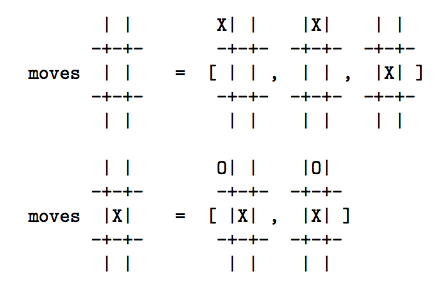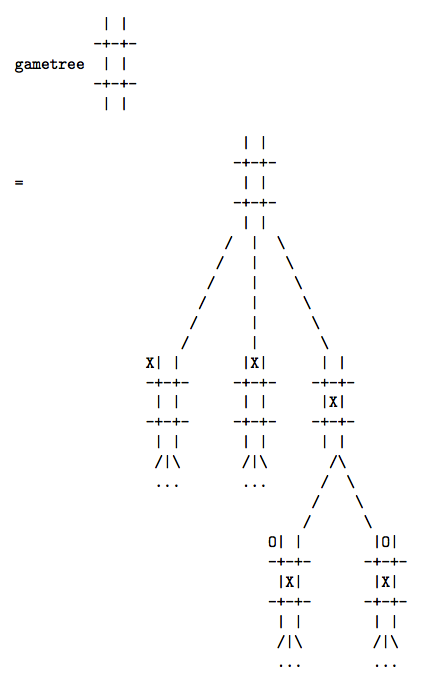Why Functional Programming Matters 函数式程序设计为什么至关重要

## 2.与结构化程序设计的相似性

“不使用goto”等等这一类特性，对于这一提升没什么作用。这些特性促进了“程序设计的小改良”，然而模块化设计却促进了“程序设计的大进化”。因此，程序员在FORTRAN或汇编语言中都可以享受结构化程序设计带来的好处，哪怕那需要一点额外的工作。

## 3.把函数粘起来

``````listof X :: = nil | cons X (listof X)
``````

``````[] 表示 nil
 表示 cons 1 nil
[1 2 3] 表示 cons 1 ( cons 2 ( cons 3 nil ))
``````

``````sum nil = 0
``````

``````sum (cons num list) = num + sum list
``````

sum nil = 0

sum (cons num list) = num + sum list

``````sum = reduce add 0
``````

``````add x y = x + y
``````

``````(reduce f x) nil = x
(reduce f x)(cons num list) = f num ((reduce f x) list)
``````

``````product = reduce multiply 1
``````

``````anytrue = reduce or false
``````

``````alltrue = reduce and true
``````

``````cons 1 (cons 2 (cons 3 nil))
``````

``````add 1 (add 2 (add 3 0)) = 6
``````

`(reduce multiply 1)`将其转换为：

``````multiply 1 (mulitiply 2 (mulitiply 3 1)) = 6
``````

``````append a b = reduce cons b a
``````

``````append [1,2] [3,4] = reduce cons [3,4] [1,2]
= (reduce cons [3,4]) (cons 1 ( cons 2 nil ))
= cons 1 ( cons 2 [3,4]))
= [1,2,3,4] （将cons替换为cons，将nil替换为[3,4]）
``````

``````doubleall = reduce doubleandcons nil
doubleandcons num list = cons ( 2*num ) list
``````

`doubleandcons`可以进一步模块化，首先分解为：

``````doubleandcons = fandcons double
double n = 2*n
fandcons f elem list = cons (f elem) list
``````

``````fandcons f = cons . f
``````

``````(f . g) h = f(g h)
``````

``````fandcons f elem
= (cons . f) elem
= cons (f elem)
``````

``````fandcons f elem list = cons (f elem) list
``````

``````doubleall = reduce (cons . double) nil
``````

``````doubleall = map double
map f = reduce (cons . f) nil
``````

``````summatrix = sum . map sum
``````

`map sum`使用函数`sum`分别计算所有行的元素之和，而后最左边的`sum`将每一行的元素之和累加起来，从而得到整个矩阵的累加和。

``````treeof X ::= node X (listof (treeof X))
``````

``````           1 o
/\
/  \
/    \
2 o     o 3
|
|
|
o 4
``````

``````node 1(cons
(node 2 nil)
(cons (node 3
(cons (node 4 nil) nil)
)
nil))
``````

``````redtree f g a (node label subtrees) = f label (redtree' f g a subtrees )
redtree' f g a (cons subtree rest) = g (redtree f g a subtree) (redtree' f g a rest)
redtree' f g a nil = a
``````

［这相当于`f`取代`node``g`取代`cons``a`取代`nil`。］

``````sumtree = redtree add add 0
``````

``````add 1
0))
= 10
``````

``````labels = redtree cons append nil
``````

``````cons 1
(append (cons 2 nil)
(append (cons 3
(append (cons 4 nil) nil))
nil))
= [1,2,3,4]
``````

``````maptree f = redtree (node . f) cons nil
``````

## 4.把程序粘起来

``````g (f input)
``````

## 4.1.牛顿－拉夫森求根法

``````a(n+1) = (a(n) + N/a(n)) / 2
``````

``````a = (a + N/a) / 2
2a = a + N/a
a = N/a
a*a = N
a = squareroot(N)
``````

``````C N IS CALLED ZN HERE SO THAT IT HAS THE RIGHT TYPE
X = A0
Y = A0 + 2.*EPS
C THE VALUE OF Y DOES NOT MATTER SO LONG AS ABS(X-Y).GT.EPS
100 IF (ABS(X-Y).LE.EPS) GOTO 200
Y = X
X = (X + ZN/X) / 2
200 CONTINUE
C THE SQUARE ROOT OF ZN IS NOW IN X
``````

［这是一段FORTRAN的程序，C代表注释行。.LE.是“Less than or Equal to”（小于或等于）的缩写，同理.GT.是“大于”的意思。]

``````next N x = (x + N/x) / 2
``````

``````[a0, f a0, f(f a0), f(f(f a0)), ...]
``````

``````repeat f a = cons a (repeat f (f a))
``````

``````repeat (next N) a0
``````

`repeat`是一个具有“无穷”输出的函数的例子——但这没关系，因为超出程序其余部分需求的近似值并不会被计算。无穷性只是潜在的：它只说明，只要有需求，就可以计算出任意数量的近似值，`repeat`本身不会强加任何限制。

``````within eps (cons a (cons b rest)) = {
= b, 如果 abs(a-b) <= eps
= within eps (cons b rest), 其他情况
}
``````

``````sqrt a0 eps N = within eps (repeat (next N) a0)
``````

``````relative eps (cons a (cons b rest)) =
= b, 如果 abs(a-b) <= eps*abs b
= relative eps (cons b rest), 其他情况
``````

［注意：`relative`里的`eps``within`里的`eps`定义是不同的！前者是绝对误差后者是相对误差！］

## 4.2.数值微分

``````easydiff f x h = (f(x+h)-f x) / h
``````

``````differentiate h0 f x = map (easydiff f x) (repeat halve h0)
halve x = x/2
``````

``````within eps (differentiate h0 f x)
``````

``````微分的精确值 ＋ 一个关于h的误差项
``````

``````a(i)   = A + B*(2**n)*(h**n)
a(i+1) = A + B*(h**n)
``````

``````A=(a(i+1)*(2**n) - a(i))/(2**n - 1)
``````

``````elimerror n (cons a (cons b rest)) =
= cons ((b*(2**n)-a)/(2**n-1)) (elimerror n (cons b rest))
``````

``````order (cons a (cons b (cons c rest))) =
= round(log2( (a-c)/(b-c) - 1 ))
round x = 最接近x的整数
log2 x  = x以2为底的对数
``````

``````improve s = elimerror (order s) s
``````

``````within eps (improve (differentiate h0 f x))
``````

`improve`只对利用一个不断减半的参数`h`计算得到的近似值序列适用。但是，如果`improve`作用于这样的序列，那么其结果也是一个这样的序列！这意味着一个近似值序列可以优化不止一次。每一次优化的过程中，都有一个不同的误差项被消除，因此优化产生的序列收敛得越来越快。因此，可以非常高效地计算导数：

``````within eps (improve (improve (improve (differentiate h0 f x))
``````

``````super s = map second (repeat improve s)
second (cons a (cons b rest)) = b
``````

`super`函数使用`repeat improve`来生成一个不断被优化的近似值的序列的序列。［就是说，生成一个序列，其中每一个元素是一个近似值序列，而这个元素是用前一个元素优化得到的。］同时，`super`提取出每个近似值序列中的第二个元素，构造出一个新的序列（已经确认，第二元素是最佳选择——它比首元更精确，而且不需要额外的计算）。这个算法的确非常复杂——更多的近似值被计算的同时，它使用了不断优化的数值方法。可以用下面的程序非常非常高效地计算导数：

``````within eps (super (differentiate h0 f x))
``````

## 4.3.数值积分

``````easyintegrate f a b = (f a + f b)*(b-a)/2
``````

``````intergrate f a b = cons (easyintergrate f a b)
(map addpair (zip (intergrate f a mid)
(intergrate f mid b)))

``````

`zip`是另一个标准的表处理函数。它读取两个列表，并返回一个有序对的列表，每个有序对由两个输入列表中对应的元素组成。从而第一对由列表一和列表二的首元组成，第二对由列表一和列表二的第二个元素组成，以此类推。`zip`可以定义为：

``````zip (cons a s) (cons b t) = cons (pair a b) (zip s t)
``````

``````intergrate f a b = interg f a b (f a) (f b)
integ f a b fa fb = cons ((fa+fb)*(b-a)/2)
(map addpair (zip (interg f a m fa fm)
(interg f m b fm fb)))

fm = f m
``````

`integrate`给出了一个不断趋近准确值的积分近似值列表，正如`differentiate`在上一小节中所做的一样。因此可以写出计算式以求出所需任意精度的积分值，如下：

``````within eps (intergrate f a b)
relative eps (integrate f a b)
``````

``````super (intergrate sin 0 4)
improve (intergrate f 0 1)

``````

（后一个序列是用于计算`pi/4`的“第八阶方法”。其中的第二个近似值只需要计算5次`f`的取值，但却具有5位准确数字。）

## 5.人工智能中的例子

``````moves: position -> listof position
````````````reptree f a = node a (map (reptree f) (f a))
``````

``````gametree p = reptree moves p
``````alpha-beta算法从一个给定的局势出发，就游戏的发展将会是有利还是不利作出判断。然而，要做到这一点，它必须能够在不考虑下一步的情况下粗略地估计某一个局势的“价值”。在后继局势不可预测时必须使用这一函数，它也可以用来对算法进行先期引导。静态估价的结果是从计算机的角度考虑的，是对该局势的前途的度量（假设在游戏中计算机与人对抗）。结果越大，局势对计算机而言越好。结果越小，局势越糟。最简单的此类函数将会，比如说，对计算机确定胜利的局势返回+1，对计算机确定失败的局势返回-1，而对其它的局势返回0。在现实中，静态估价函数会衡量各种使局势“看上去不错”的因素。例如，具体的好处，以及象棋中对中心的控制。假定有这样一个函数：

``````static: position -> number
``````

［所谓“真值”（true value），可能是我翻译得不好，此处理解为类似“真正的价值”的意思吧，是一个量度，不是逻辑学里的0和1哦。］

``````maximise (node n sub) = max (map minimise sub)
minimise (node n sub) = min (map maximise sub)
``````

``````maximise (node n nil) = n
maximise (node n sub) = max (map minimise sub)
maximise (node n nil) = n
maximise (node n sub) = min (map minimise sub)
``````

``````evaluate = maximise . maptree static . gametree
``````

``````prune 0 (node a x) = node a nil
prune n (node a x) = node a (map (prune (n-1)) x)
``````

`(prune n)`取一棵树作为参数并“剪去”与根结点的距离超过n的所有结点。如果一棵博弈树被剪枝，那么将强制`maximise`对深度为`n`的结点执行静态估价而不是进一步递归。因此`evaluate`可以被定义为：

``````evaluate = maximise . maptree static . prune 5 . gametree
``````

``````prune 5 . gametree
``````

``````maptree static . prune 5 . gametree
``````

``````          max
/ \
/   \
/     \
/       \
min       min
/ \       / \
/   \     /   \
1     2   0     ?
``````

``````maximise = max . maximise'
``````

`minimise`可以用类似的方法分解。由于`maximise``minimise`是完全对称的，故我们将只讨论`maximise`，而假定`minimise`也照此处理。）一旦这样分解之后，`maximise`可以使用`minimise'`来发现`minimise`将对哪些数字求最小值，并且不再使用`minimise`本身。而后便可以在不查看某些数字的情况下便将它们丢弃。由于惰性求值的存在，如果`maxmise`并不会查看所有的数字列表，那么一部分列表将不会被计算，这是对计算机时间的潜在节约。

`max``maximise`中“约分出来”是很简单的，得到：

``````maximise' (node n nil) = cons n nil
maximise' (node n l) = map minimise l
= map (min . minimise') l
= map min (map minimise' l)
= mapmin (map minimise' l)

``````

``````mapmin (cons nums rest) =
= cons (min nums) (omit (min nums) rest)
``````

``````omit pot nil = nil
omit pot (cons nums rest) =
= omit pot rest, if minleq nums pot
= cons (min nums) (omit (min nums) rest), otherwise
``````

`minleq`以一个数字列表和一个潜在最大值为参数，如果列表的最小值小于或等于潜在最大值就返回真。要完成这一工作，它并不需要扫描整个列表！如果列表中有任意一个元素小于或等于潜在最大值，那么列表的最小值肯定也是如此。该特别元素之后的所有元素都是无关紧要的——它们就像是上面例子中的问号一样。因此`minleq`可以被定义为：

``````minleq nil pot = false
minleq (cons num rest) port = true, if numn2
``````

``````evaluate = max . maximise' . highfirst . maptree static . prune 8 . gametree
``````

``````taketree n = redtree (nodett n) cons nil
nodett n label sub = node label (take n sub)
``````

`taketree`将树上所有的结点替换为最多有n个子结点的结点，它使用了函数`(take n)`，而该函数返回列表的前n个元素（如果列表比n短，那么返回的元素就少一些）。

``````prune 0 (node pos sub) = node pos (map (prune 0) sub), if dynamic pos
``````

## 参考文献

• [AS86] H.Abelson,G.J.Sussman. 计算机程序的构造与解释. 麻省理工学院出版社,波士顿,1986
• [Hug89] J.Hughes. 函数式程序设计为什么至关重要. 计算机月刊,32(2),1989
• [Hug90] John Hughes. 函数式程序设计为什么至关重要. D.Turner主编,函数式编程的研究主题. Addison Wesley,1990
• [MTH90] R.Milner,M.Tofte,R.Harper. Standard ML的定义. 麻省理工学院出版社,1990